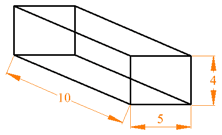# 方程与公式

## 方程是什么？

 x + 2 = 6

## 公式是什么？

### 例子：长方体体积的公式是：

V = lwh

V 是体积， l 是长， w 是宽，h 是高V = 10 × 5 × 4 = 200

 x = 2y - 7 公式 （显示 x 和 y的关系） a2 + b2 = c2 公式 （显示 a、b 和 c的关系） x/2 + 7 = 0 不是公式（只是方程）

lwh

s = ut + ½ at2

"s" 是公式的主词

## 转换主词

 开始： V = lwh 每边除以 h： V/h = lw 每边除以 l： V/(hl) = w 两边互换： w = V/(hl)

w = V/(hl)

w = 12m3 / (2m × 2m) = 12/4 = 3m